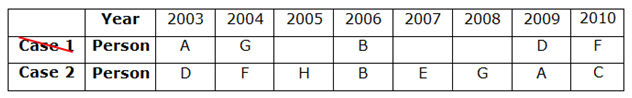# SBI Clerk Prelims Reasoning Ability Questions 2021 (Day-86)

Dear Aspirants, Our IBPS Guide team is providing new series of Reasoning Questions for SBI Clerk Prelims 2021 so the aspirants can practice it on a daily basis. These questions are framed by our skilled experts after understanding your needs thoroughly. Aspirants can practice these new series questions daily to familiarize with the exact exam pattern and make your preparation effective.

Start Quiz

Direction sense

Direction (1-3): Study the following information carefully and answer the below questions

Point P is 2m north of Point Q. Point Z is 9m west of Point Q. Point L is 15m east of Point M which is 20m south of Point Z. Point R is 3m south of Point L. Point T is west of point R and South of Point P.

1) How far and what is the direction of Q with respect to T?

A.21m towards the south

B.20m towards the south

C.23m towards the north

D.22m towards the north

E.None of the above

2) What is the shortest distance between Point L and Point Z?

A.21m

B.24m

C.20m

D.23m

E.25m

3) What is the shortest distance between T and R?

A.6m

B.15m

C.7m

D.9m

E.None of the above

Inequality

Direction (4-5): In these questions, the relationship between different elements is shown in the statements. These statements are followed by two conclusions. Find the conclusion which logically follows.

(a) If only conclusion I follow.

(b) If only conclusion II follows.

(c) If either conclusion I or II follows.

(d) If neither conclusion I nor II follows.

(e) If both conclusion I and II follow.

4) Statements:

P ≥ N = G > T < K > L

Conclusion:

I). G < K

II). P > T

5) Statements:

Q > K > L ≤ C = H > I

Conclusion:

I). K > I

II). H > L

Year puzzle

Direction (6-10): Answer the questions based on the information given below.

Eight persons viz. A, B, C, D, E, F, G and H were born on eight consecutive years starting from 2003 to 2010, but not necessarily in the same order. The consecutive alphabetically named persons don’t born consecutively.

G was born in a leap year. Only four persons were born between G and D Who was born immediately before F. The number of persons born before F is the same as the number of persons born after A. Only two persons were born between A and B.

6) Who among the following person was born in 2005?

A.E

B.F

C.C

D.H

E.Cannot be determined

7) How many persons were born between B and C?

A.One

B.Two

C.Three

D.More than three

E.None

#### 8) If all the persons were born in alphabetical order, then how many persons remain unchanged in their positions?

A.One

B.Two

C.Three

D.More than three

E.None

9) Who among the following person was born two persons before G?

A.E

B.B

C.F

D.No such person

E.None of these

10) In which of the following year does C was born?

A.2007

B.2009

C.2005

D.2003

E.2010

Directions (1-3) :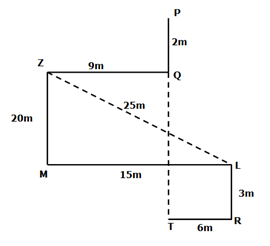202+152=ZL2

√(202+152)=√625=25m

Directions (6-10) :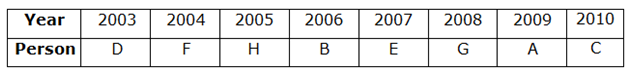1) G was born in a leap year. Only four persons were born between G and D Who was born immediately before F.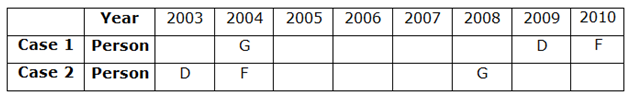2) The number of persons born before F is the same as the number of persons born after A. Only two persons were born between A and B.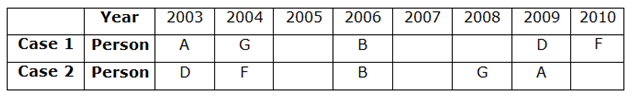3) The consecutive alphabetically named persons don’t born consecutively. Hence, case 1 gets eliminated.